## Back Propagation Algorithm in Neural Network

In an artificial neural network, the values of weights and biases are randomly initialized. Due to random initialization, the neural network probably has errors in giving the correct output. We need to reduce error values as much as possible. So, for reducing these error values, we need a mechanism which can compare the desired output of the neural network with the network’s output that consist of errors and adjust its weights and biases such that it gets closer to the desired output after each iteration. For this, we train the network such that it back propagates and updates the weights and biases. This is the concept of back propagation algorithm.

Below are the steps that an artificial neural network follows to gain maximum accuracy and minimize error values:

## Understanding Deep Learning

1. Parameter Initialization
2. Feedforward Propagation
3. BackpropagationWe will look into all these steps, but mainly we will focus on back propagation algorithm.

### Parameter Initialization

In this, parameters, i.e., weights and biases, associated with an artificial neuron are randomly initialized. After receiving the input, the network feed forwards the input and it makes associations with weights and biases to give the output. The output associated to those random values is most probably not correct. So, next, we will see feedforward propagation.

Want to become master in Artificial Intelligence, check out this Artificial Intelligence Course!

### Feedforward propagation

After initialization, when the input is given to the input layer, it propagates the input into hidden units at each layer. The nodes here do their job without being aware whether results produced are accurate or not (i.e., they don’t re-adjust according to the results produced). Then, finally, the output is produced at the output layer. This is called feedforward propagation.

### Back propagation in Neural Networks

The principle behind back propagation algorithm is to reduce the error values in randomly allocated weights and biases such that it produces the correct output. The system is trained in the supervised learning method, where the error between the system’s output and a known expected output is presented to the system and used to modify its internal state. We need to update the weights such that we get the global loss minimum. This is how back propagation in neural networks works.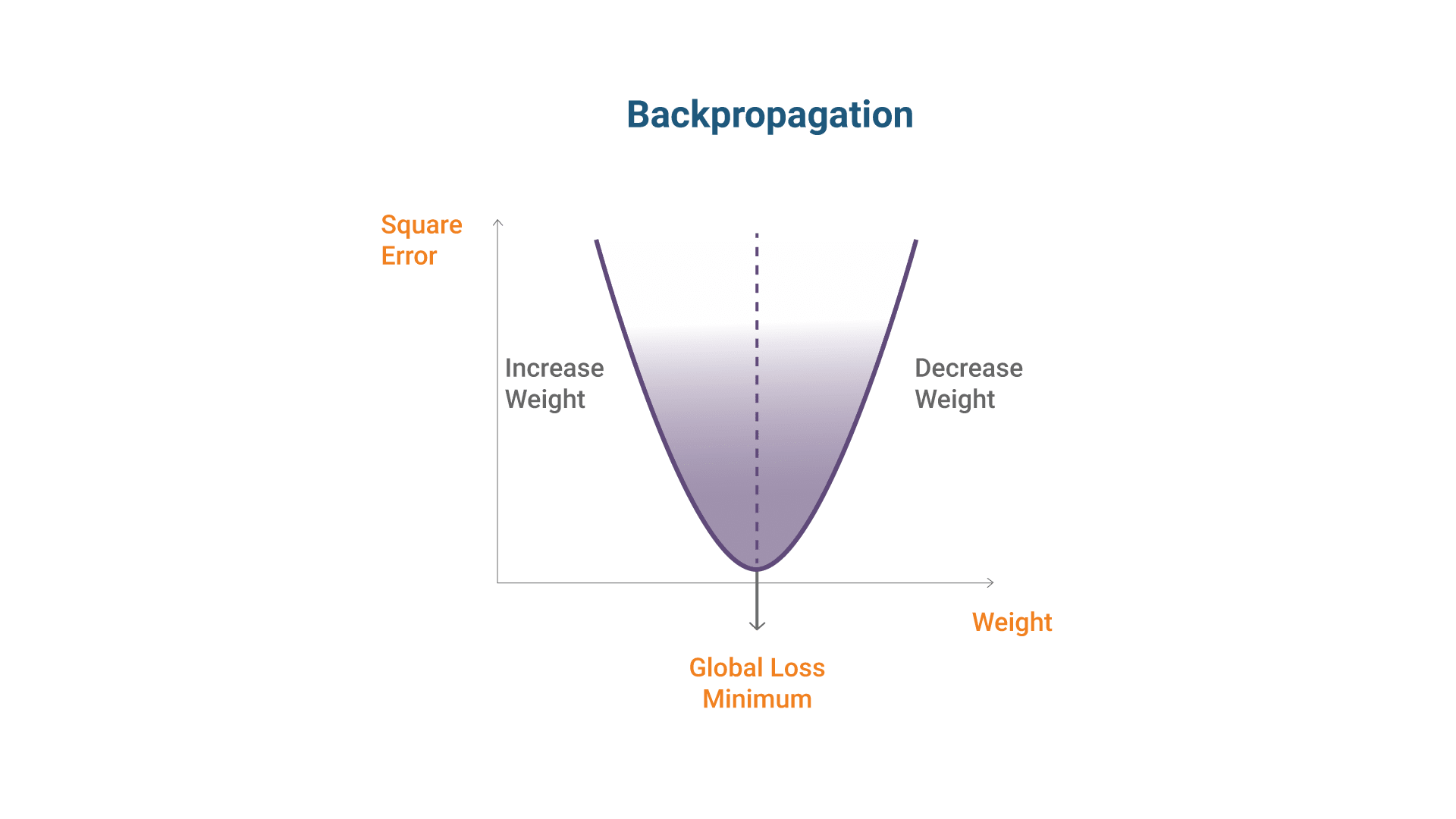When the gradient is negative, increase in weight decreases the error.

When the gradient is positive, decrease in weight decreases the error.

## Working of Back Propagation Algorithm

How does back propagation algorithm work?

The goal of back propagation algorithm is to optimize the weights so that the neural network can learn how to correctly map arbitrary inputs to outputs. Here, we will understand the complete scenario of back propagation in neural networks with help of a single training set.

Go through the Artificial Intelligence Course in Sydney to get clear understanding of Weak AI and Strong AI.

In order to have some numbers to work with, here are initial weights, biases, and training input and output.

```Inputs(i1): 0.05                          Output (o1): 0.01

Inputs(i2): 0.10                          Output(o2):0.99```

### Step 1: The Forward Pass: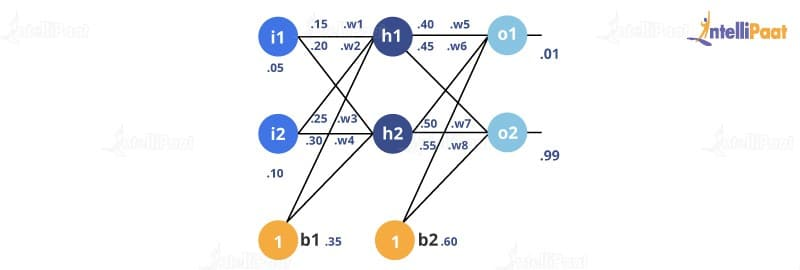The total net input for h1: The net input for h1 (the next layer) is calculated as the sum of the product of each weight value and the corresponding input value and, finally, a bias value added to it.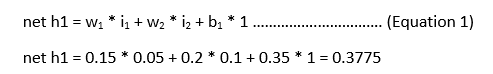The output for h1: The output for h1 is calculated by applying sigmoid function to the net input Of h1.

The sigmoid function pumps the values for which it is used in the range, 0 to 1.

It is used for models where we have to predict the probability. Since the probability of any event lies between 0 and 1, the sigmoid function is the right choice.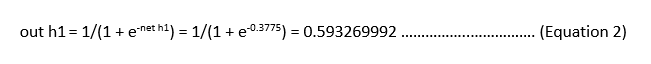Carrying out the same process for h2:

`out h2 = 0.596884378`

The output for o1: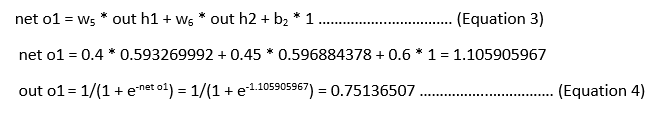Carrying out the same process for o2:

`out o2 = 0.772928465`

### Calculating the Total error:

We can now calculate the error for each output neuron using the squared error function and sum them up to get the total error: E total = Ʃ1/2(target – output)2

The target output for o1 is 0.01, but the neural network output is 0.75136507; therefore, its error is:

`E o1 = 1/2(target o1 - out o1)2 = 1/2(0.01 - 0.75136507)2 = 0.27481108 ……………..……………. (Equation 5)`

By repeating this process for o2 (remembering that the target is 0.99), we get:

`E o2 = 0.023560026`

Then, the total error for the neural network is the sum of these errors:

`E total = E o1 + E o2 = 0.274811083 + 0.023560026 = 0.298371109`

### Step 2: Backward Propagation:

Our goal with back propagation algorithm is to update each weight in the network so that the actual output is closer to the target output, thereby minimizing the error for each output neuron and the network as a whole.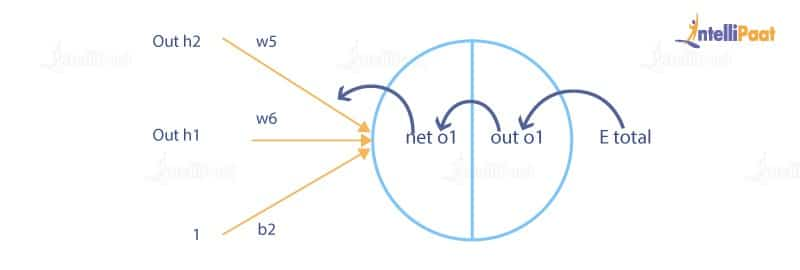Consider w5; we will calculate the rate of change of error w.r.t the change in the weight w5: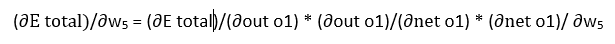Since we are propagating backward, the first thing we need to do is to calculate the change in total errors w.r.t the outputs o1 and o2: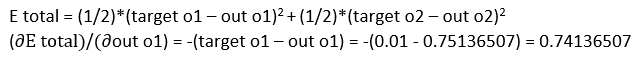Now, we will propagate further backward and calculate the change in the output o1 w.r.t to its total net input: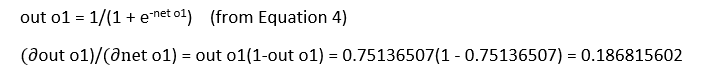How much does the total net input of o1 change w.r.t w5?Putting all values together and calculating the updated weight value: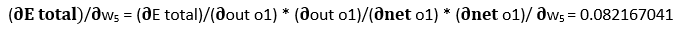Let’s calculate the updated value of w5.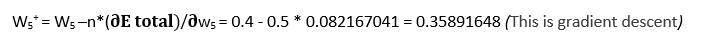We can repeat this process to get the new weights w6, w7, and w8.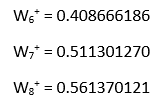We perform the actual updates in the neural network after we have the new weights leading into the hidden layer neurons.

We’ll continue the backward pass by calculating new values for w1, w2, w3, and w4:

Starting with w1: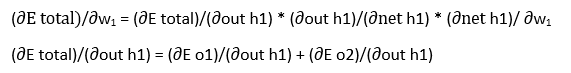We’re going to use a similar process as we did for the output layer, but slightly different to account for the fact that the output of each hidden layer neuron contributes to the final output. Thus, we need to take Eo1 and Eo2 into consideration.

We can visualize it as below:Starting with h1: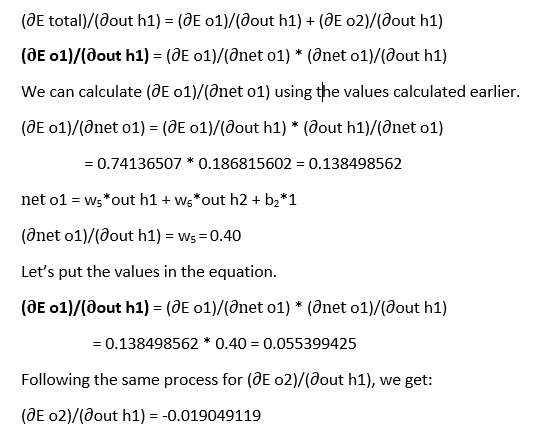We can calculate: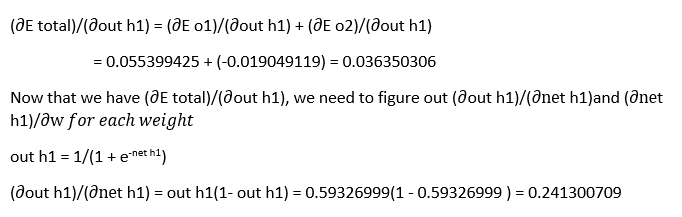We will calculate the partial derivative of the total net input of h1 w.r.t w1 the same way as we did for the output neuron.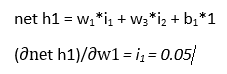Let’s put it all together.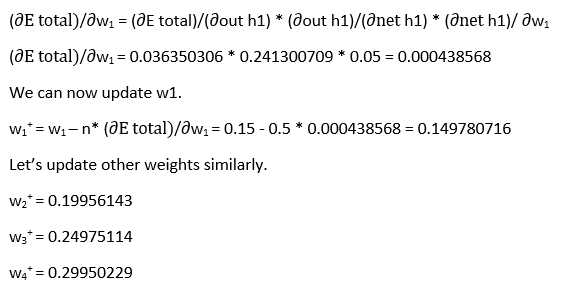• When we fed forward 0.05 and 0.1 inputs originally, the error on the network was 0.298371109.
• After the first round of backpropagation, the total error is now down to 0.291027924.It might not seem like much, but after repeating this process 10,000 times, for example, the error plummets to 0.0000351085. At this point, when we feedforward 0.05 and 0.1, the two output neurons will generate 0.015912196 (vs. 0.01 target) and 0.984065734 (vs. 0.99 target).

Now, in this back propagation algorithm blog, let’s go ahead and comprehensively understand “Gradient Descent” optimization.

Prepare yourself for the Artificial Intelligence Interview questions and answers Now!

• Gradient descent is by far the most popular optimization strategy used in Machine Learning and Deep Learning at the moment. It is used while training our model, can be combined with every algorithm, and is easy to understand and implement.
• Gradient measures how much the output of a function changes if we change the inputs a little.
• We can also think of a gradient as the slope of a function. The higher the gradient, the steeper the slope and the faster the model learns.
•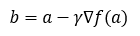where,

b = next value

a = current value

‘−’ refers to the minimization part of the gradient descent.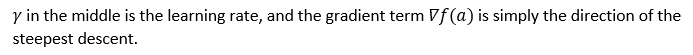• This formula basically tells us the next position where we need to go, which is the direction of the steepest descent.
• Gradient descent can be thought of as climbing down to the bottom of a valley, instead of as climbing up a hill. This is because it is a minimization algorithm that minimizes a given function.
• Let’s consider the graph below where we need to find the values of w and b that correspond to the minimum cost function (marked with a red arrow).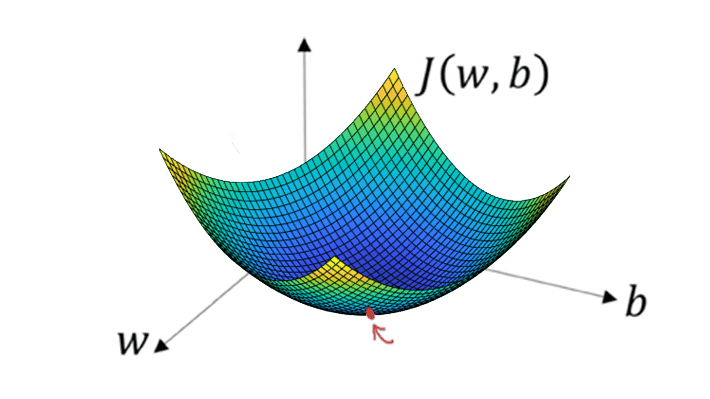• To start with finding the right values, we initialize the values of w and b with some random numbers, and gradient descent starts at that point (somewhere around the top).
• Then, it takes one step after the other in the steepest downside direction (e.g., from top to bottom) till it reaches the point where the cost function is as small as possible.

### Batches

• The total number of training examples present in a single batch is referred to as the batch size.
• Since we can’t pass the entire dataset into the neural net at once, we divide the dataset into number of batches or sets or parts.

Moving ahead in this blog on “Back Propagation Algorithm”, we will look at the types of gradient descent.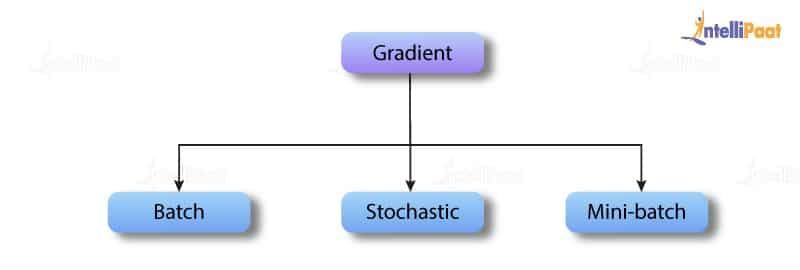In batch gradient descent, we use the complete dataset available to compute the gradient of the cost function. Batch gradient descent is very slow because we need to calculate the gradient on the complete dataset to perform just one update, and if the dataset is large then it will be a difficult task.

1. Cost function is calculated after the initialization of parameters.
2. It reads all the records into memory from the disk.
3. After calculating sigma for one iteration, we move one step further, and repeat the process.

It is a widely used algorithm that makes faster and accurate results. The dataset, here, is clustered into small groups of ‘n’ training datasets. It is faster because it does not use the complete dataset. In every iteration, we use a batch of ‘n’ training datasets to compute the gradient of the cost function. It reduces the variance of the parameter updates, which can lead to more stable convergence. It can also make use of a highly optimized matrix that makes computing of the gradient very efficient.

Go through this AI Course in London to get a clear understanding of Artificial Intelligence!

We use stochastic gradient descent for faster computation. The first step is to randomize the complete dataset. Then, we use only one training example in every iteration to calculate the gradient of the cost function for updating every parameter. It is faster for larger datasets also because it uses only one training example in each iteration.

We understood all the basic concepts and working of back propagation algorithm through this blog. Now, we know that back propagation algorithm is the heart of a neural network.

### Watch this Artificial Intelligence Project

Course Schedule

Name Date
Data Science Course 2021-10-23 2021-10-24
(Sat-Sun) Weekend batch
View Details
Data Science Course 2021-10-30 2021-10-31
(Sat-Sun) Weekend batch
View Details
Data Science Course 2021-11-06 2021-11-07
(Sat-Sun) Weekend batch
View Details

Browse Categories